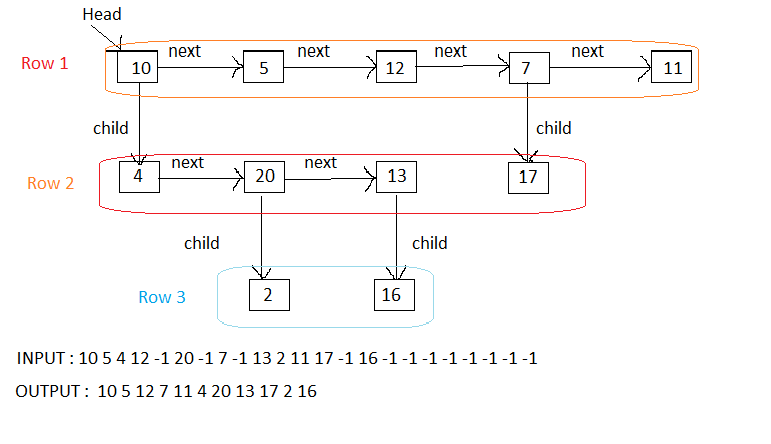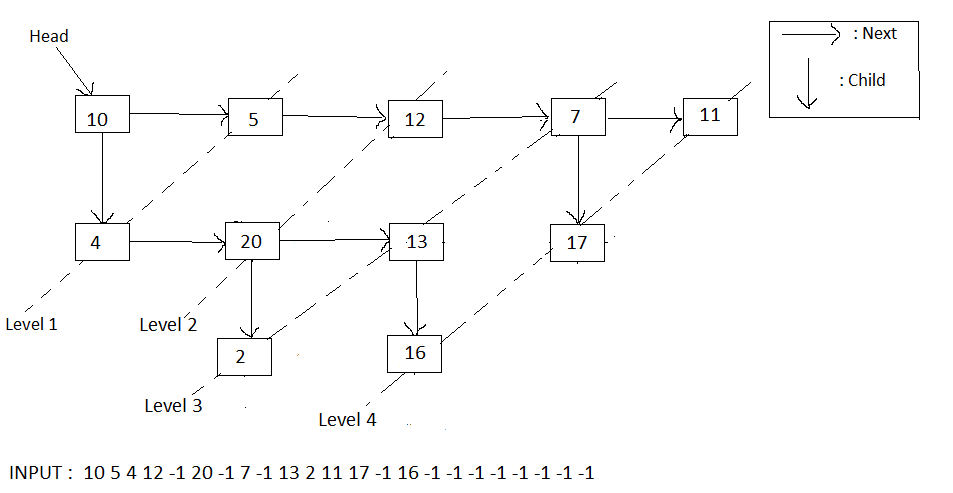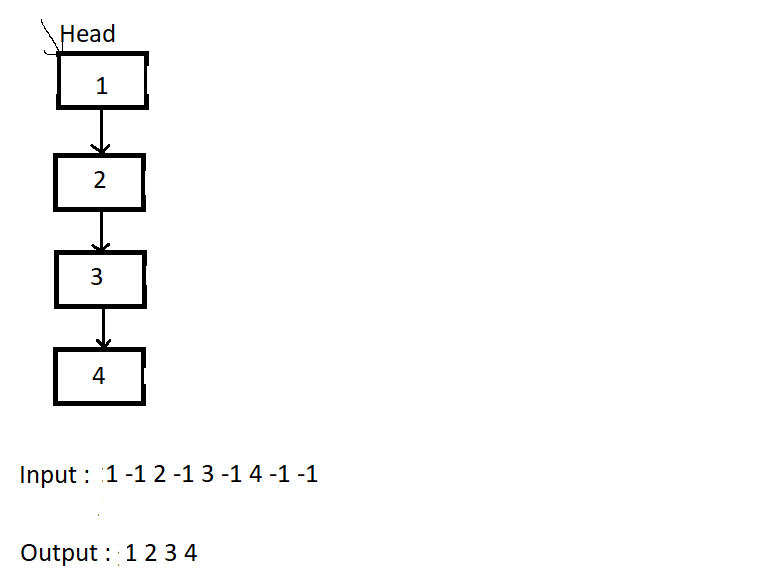8

# Flatten The Multi-Level Linked List

Difficulty: MEDIUMContributed By
Ambuj verma
Avg. time to solve
10 min
Success Rate
80%

Problem Statement
Suggest Edit

#### Example:#### Flatten :

``````All the different rows are merged into a single row.
``````
##### Input Format:
``````The first line contains an integer 'T' which denotes the number of test cases or queries to be run. Then the test cases follow.

The first line of each test case contains the elements of the multi-level linked-list in the level order form. The line consists of values of nodes separated by a single space. In case a node(next or child pointer) is null, we take -1 in its place.

For example, the input for the multi-level linked-list depicted in the below image would be :
````````````10
5 4
12 -1 20 -1
7 -1 13 2
11 17 -1 16 -1 -1
-1 -1 -1 -1 -1 -1
``````

#### Explanation :

``````Level 0 :

Level 1 :
Next pointer of 10 = 5
Child pointer of 10 = 4

Level 2 :
Next pointer of 5 = 12
Child pointer of 5 = null (-1)
Next pointer of 4 = 20
Child pointer of 4 = null(-1)

Level 3 :
Next pointer of 12= 7
Child pointer of 12 = null(-1)
Next pointer of 20 = 13
Child pointer of 20 = 2

Level 4 :
Next pointer of 7 = 11
Child pointer of 7 = 17
Next pointer of 13 = null(-1)
Child pointer of 13 = 16
Next pointer of 2 = null(-1)
Child pointer of 2 = null(-1)

The first not-null node (of the previous level) is treated as the parent of the first two nodes of the current level(next and child pointers). The second not-null node (of the previous level) is treated as the parent node for the next two nodes of the current level and so on.

The input ends when all nodes at the last level are null (-1).
``````
##### Note :
``````The above format was just to provide clarity on how the input is formed for a given multi-level linked list.

The sequence will be put together in a single line separated by a single space. Hence, for the above-depicted multi-level linked list, the input will be given as:

10 5 4 12 -1 20 -1 7 -1 13 2 11 17 -1 16 -1 -1 -1 -1 -1 -1 -1 -1
``````
##### Output format :
``````For each test case, print the flattened linked-list of the multi-level linked-list in a single line.
``````
##### Note:
``````You do not need to print anything, it has already been taken care of. Just implement the given function.
``````
##### Constraints:
``````1 <= T <= 10
0 <= N <= 10^4
1 <= data <= 10^9

Time Limit : 1 sec
``````
##### Sample Input 1 :
``````1
1 -1 2 -1 3 -1 4 -1 -1
``````
##### Sample Output 1 :
``````1 2 3 4
``````
##### Explanation Of the Sample Input 1 :
``````The given multi-level linked-list will be represented as shown below
``````##### Sample Input 2 :
``````1
10 5 4 12 -1 20 -1 7 -1 13 2 11 17 -1 16 -1 -1 -1 -1 6 9 -1 3 -1 -1 8 19 -1 -1 -1 -1 15 -1 -1 -1
``````
##### Sample Output 2 :
``````10 5 12 7 11 4 20 13 17 6 2 16 9 8 3 19 15
``````Console